# 深度學習煉丹-資料預處理和增強

## 前言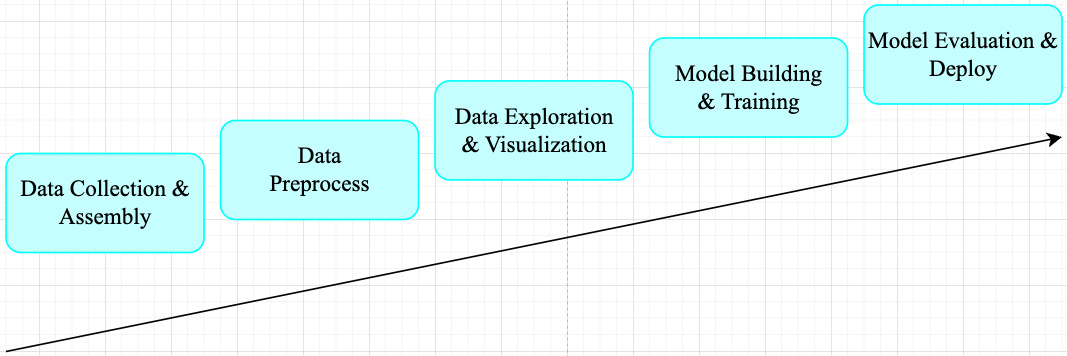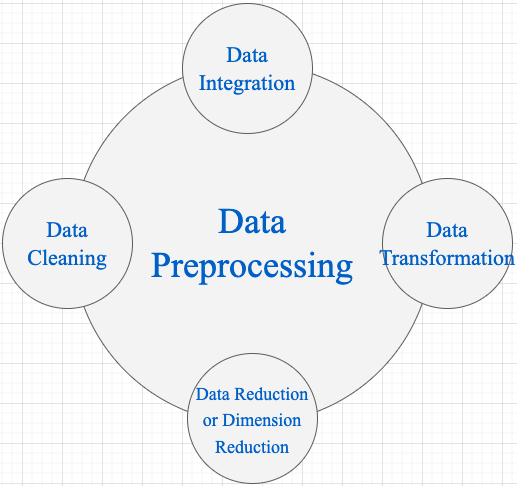1，Data Cleaning 資料清理是資料預處理步驟的一部分，通過填充缺失值、平滑噪聲資料、解決不一致和刪除​​異常值來清理資料。 2，Data Integration 用於將存在於多個源中的資料合併到一個更大的資料儲存中，如資料倉庫。例如，將來自多個醫療節點的影象整合起來，形成一個更大的資料庫。 3，在完成 Data Cleaning 後，我們需要通過使用下述資料轉換策略更改資料的值、結構或格式。 - Generalization: 使用概念層次結構將低階或粒度資料轉換為高階資訊。例如將城市等地址中的原始資料轉化為國家等更高層次的資訊。 - Normalization: 目的是將數字屬性按比例放大或縮小以適合指定範圍。Normalization 常見方法: - Min-max normalization - Z-Score normalization - Decimal scaling normalization

4，Data Reduction 資料倉庫中資料集的大小可能太大而無法通過資料分析和資料探勘演算法進行處理。一種可能的解決方案是獲得資料集的縮減表示，該資料集的體積要小得多，但會產生相同質量的分析結果。常見的資料縮減策略如下: - Data cube aggregation - Dimensionality reduction: 降維技術用於執行特徵提取。資料集的維度是指資料的屬性或個體特徵。該技術旨在減少我們在機器學習演算法中考慮的冗餘特徵的數量。降維可以使用主成分分析（PCA）等技術來完成。 - Data compression: 通過使用編碼技術，資料的大小可以顯著減小。 - Discretization: 資料離散化用於將具有連續性的屬性劃分為具有區間的資料。這樣做是因為連續特徵往往與目標變數相關的可能性較小。例如，屬性年齡可以離散化為 18 歲以下、18-44 歲、44-60 歲、60 歲以上等區間。

## 一，Normalization 概述

### 1.1，Normalization 定義

Normalization 操作被用於對資料屬性進行縮放，使其落在較小的範圍之內（即變化到某個固定區間中），比如 [-1,1] 和 [0, 1]，簡單理解就是特徵縮放過程。很多機器學習演算法都受益於 Normalization 操作，比如:

• 通常對分類演算法有用。
• 在梯度下降等機器學習演算法的核心中使用的優化演算法很有用。
• 對於加權輸入的演算法（如迴歸和神經網路）以及使用距離度量的演算法（如 K 最近鄰）也很有用。

### 1.2，什麼情況需要 Normalization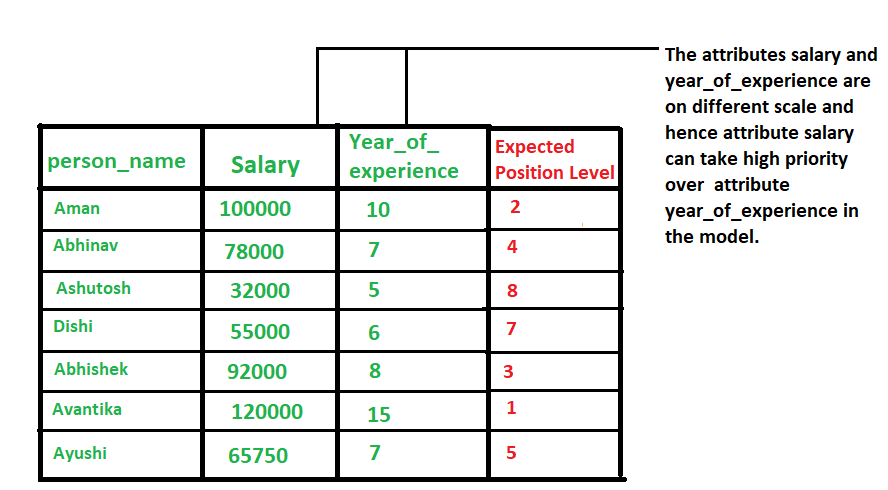### 1.3，Data Normalization 方法

1，z-Score Normalization

zero-mean Normalization，有時也稱為 standardization，將資料特徵縮放成均值為 0，方差為 1 的分佈，對應公式:

$${x}' = \frac{x-mean(x)}{\sigma}$$

2，Min-Max Normalization

$${x}' = \frac{x - min(x)}{max(x) - min(x)}$$

### 1.4，示例程式碼

1，以下是使用 Python 和 Numpy 庫實現 Min-Max Normalization 的示例程式碼：

python

# 匯入必要的庫

import numpy as np

# 定義資料集

X = np.array([[1, 2, 3], [4, 5, 6], [7, 8, 9]])

# 計算資料集的最小值和最大值

Xmin = X.min() Xmax = X.max()

# 計算最小-最大規範化

X_norm = (X - Xmin) / (Xmax - Xmin)

# 列印結果

print(X_norm)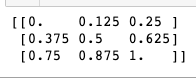## 二，normalize images

### 2.1，影象 normalization 定義

1，以單張影象的 zero-mean Normalization 為例，它使得影象的均值和標準差分別變為 0.01.0。因為是多維資料，與純表格資料不同，它首先需要從每個輸入通道中減去通道平均值，然後將結果除以通道標準差。因此可定義兩種 normalization 形式如下所示:

shell

# min-max Normalization

output[channel] = (input[channel] - min[channel]) / (max[channel] - min[channel])

# zero-mean Normalization

output[channel] = (input[channel] - mean[channel]) / std[channel] 

### 2.3，PyTorch 實踐影象 normalization

Pytorch 框架中，影象變換（image transformation）是指將影象畫素的原始值改變為新值的過程。其中常見的 transformation 操作是使用 torchvision.transforms.ToTensor() 方法將影象變換為 Pytorch 張量（tensor），它實現了將畫素範圍為 [0, 255] 的 PIL 影象轉換為形狀為（C,H,W）且範圍為 [0.0, 1.0] 的 Pytorch FloatTensor。另外，torchvision.transforms.normalize() 方法實現了逐 channel 的對影象進行標準化（均值變為 0，標準差變為 1）。總結如下:

• min-max Normalization: 對應 torchvision.transforms.ToTensor() 方法
• zero-mean Normalization: 對應 torchvision.transforms.Normalize() 方法，利用用均值和標準差對張量影象進行 zero-mean Normalization。

ToTensor() 函式的語法如下: shell """ Convert aPIL Imageornumpy.ndarray to tensor. Converts a PIL Image or numpy.ndarray (H x W x C) in the range [0, 255] to a torch.FloatTensor of shape (C x H x W) in the range [0.0, 1.0].

Args: pic (PIL Image or numpy.ndarray): Image to be converted to tensor. Returns: Tensor: Converted image. """ 

Normalize() 函式的語法如下:

shell Syntax: torchvision.transforms.Normalize()

Parameter: * mean: Sequence of means for each channel. * std: Sequence of standard deviations for each channel. * inplace: Bool to make this operation in-place. Returns: Normalized Tensor image. 

# import necessary libraries

from PIL import Image import matplotlib.pyplot as plt import numpy as np import torchvision.transforms as transforms import matplotlib.pyplot as plt

def show_images(imgs, num_rows, num_cols, titles=None, scale=8.5): """Plot a list of images.

Defined in :numref:sec_utils"""
figsize = (num_cols * scale, num_rows * scale)
_, axes = plt.subplots(num_rows, num_cols, figsize=figsize)
axes = axes.flatten()
for i, (ax, img) in enumerate(zip(axes, imgs)):
try:
img = np.array(img)
except:
pass
ax.imshow(img)
ax.axes.get_xaxis().set_visible(False)
ax.axes.get_yaxis().set_visible(False)
if titles:
ax.set_title(titles[i])
return axes


def normalize_image(image_path): img = Image.open(img_path) # load the image # 1, use ToTensor function transform = transforms.Compose([ transforms.ToTensor() ]) img_tensor = transform(img) # transform the pIL image to tensor # 2, calculate mean and std by tensor's attributes mean, std = img_tensor.mean([1,2]), img_tensor.std([1,2]) # 3, use Normalize function transform_norm = transforms.Compose([ transforms.ToTensor(), transforms.Normalize(mean, std) ]) img_normalized = transform_norm(img) # get normalized image

img_np = np.array(img) # convert PIL image to numpy array
# print array‘s shape mean and std
print(img_np.shape) # (height, width, channel), (768, 1024, 3)
print("mean and std before normalize:")
print("Mean of the image:", mean)
print("Std of the image:", std)
return img_np, img_tensor, img_normalized


def convert_tensor_np(tensor): img_arr = np.array(tensor) img_tr = img_arr.transpose(1, 2, 0) return img_tr

if name == 'main': img_path = 'Koalainputimage.jpeg' img_np, img_tensor, img_normalized = normalize_image(img_path) # transpose tensor to numpy array and shape of (3,,) to shape of (,,3) img_normalized1 = convert_tensor_np(img_tensor) img_normalized2 = convert_tensor_np(img_normalized) show_images([img_np, img_normalized1, img_normalized2], 1, 3, titles=["orignal","min-max normalization", "zero-mean normalization"]) 

1，程式輸出和兩種 normalization 操作效果視覺化對比圖如下所示: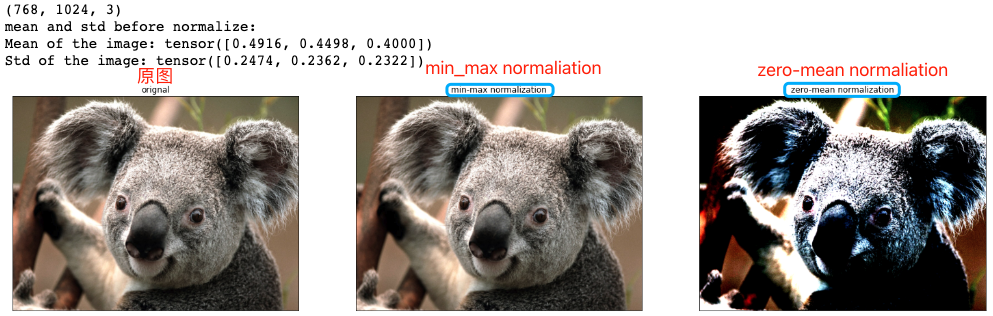2，原圖和兩種 normalization 操作後的影象畫素值分佈視覺化對比圖如下所示: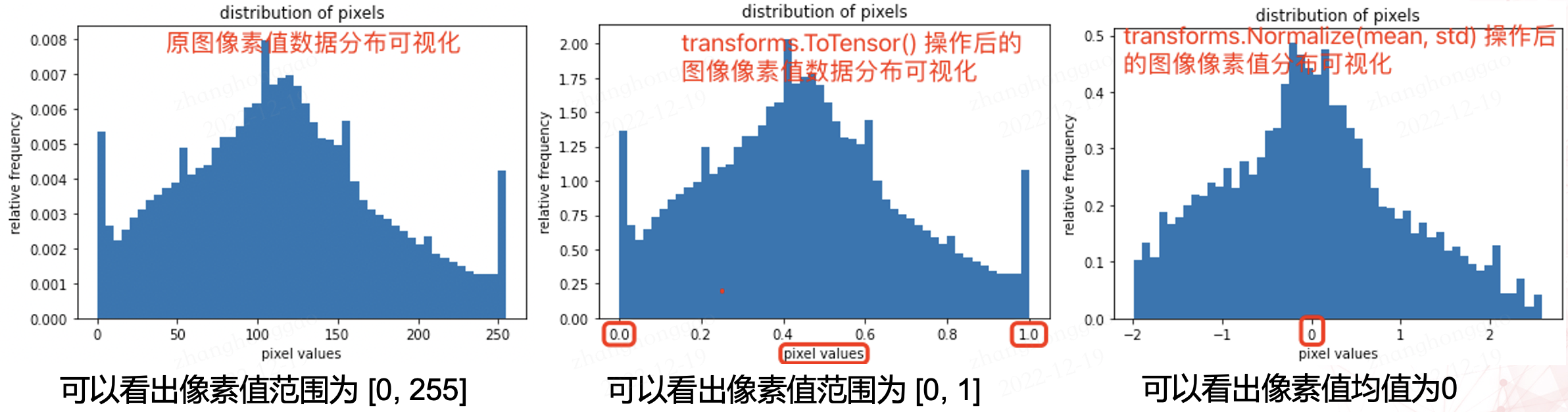python

# plot the pixel values

plt.hist(img_np.ravel(), bins=50, density=True) plt.xlabel("pixel values") plt.ylabel("relative frequency") plt.title("distribution of pixels") 

## 三，資料增強(擴增)

HSV 表達彩色影象的方式由三個部分組成： Hue（色調、色相） Saturation（飽和度、色彩純淨度） Value（明度）

### 3.1，opencv 影象處理

#### 影象的幾何變換

OpenCV 提供的幾何變換函式如下所示:

1，拓展縮放: 拓展縮放，改變影象的尺寸大小

cv2.resize(): 。常用的引數有設定影象尺寸、縮放因子和插值方法。

2，平移: 將物件換一個位置。

cv2.warpAffine(): 函式第一個引數是原影象，第二個引數是移動矩陣，第三個引數是輸出影象大小 (width,height)。舉例，如果要沿 $(x，y)$ 方向移動，移動的距離是 $(tx ，ty)$，則以下面的方式構建移動矩陣: $$\begin{bmatrix} 1 & 0 & t_x\ 0 & 1 & t_y \end{bmatrix}$$

3，旋轉: 對一個影象旋轉角度 $\theta$。

getRotationMatrix2D 函式第一個引數為旋轉中心，第二個為旋轉角度，第三個為旋轉後的縮放因子

4，放射變換（也叫平面變換/徑向變換）: 在仿射變換中，原圖中所有的平行線在結果影象中依舊平行。

5，透視變換（也叫空間變換）: 轉換之後，直線仍是直線。

python import cv2 import matplotlib.pyplot as plt from PIL import Image import numpy as np

# Some Geometric Transformation of Images

class GeometricTransAug(object): def init(self, image_path): img = Image.open(image_path) # load the image self.img_np = np.array(img) # convert PIL image to numpy array self.rows, self.cols, self.ch = self.img_np.shape self.geometry_trans_aug_visual(self.img_np)

def resize_aug(self, img_np):
# 直接設定了縮放因子, 縮放原大小的2倍
res = cv2.resize(img_np, None, fx=2, fy=2, interpolation = cv2.INTER_CUBIC)
return res

def warpAffine_aug(self, img_np):
# 先構建轉換矩陣, 將影象畫素點整體進行(100,50)位移：
M = np.float32([[1,0,100],[0,1,50]])
res = cv2.warpAffine(img_np, M,(self.cols, self.rows))
return res

def rotation_aug(self, img_np):
rows, cols, ch = img_np.shape
# 先構建轉換矩陣,影象相對於中心旋轉90度而不進行任何縮放。
M = cv2.getRotationMatrix2D((self.cols/2, self.rows/2), 90, 1)
res = cv2.warpAffine(img_np, M, (self.cols, self.rows))
return res

# 仿射變換需要從原影象中找到三個點以及他們在輸出影象中的位置
pts1 = np.float32([[50,50],[200,50],[50,200]])
pts2 = np.float32([[10,100],[200,50],[100,250]])
# 通過 getAffineTransform 建立一個 2x3 的轉換矩陣
M = cv2.getAffineTransform(pts1,pts2)

res = cv2.warpAffine(img_np, M, dsize = (self.cols, self.rows))
return res

def perspective_trans_aug(self, img_np):
# 透視變換需要一個 3x3 變換矩陣
pts1 = np.float32([[56,65],[368,52],[28,387],[389,390]])
pts2 = np.float32([[0,0],[300,0],[0,300],[300,300]])

M = cv2.getPerspectiveTransform(pts1,pts2)
# dsize: size of the output image.
res = cv2.warpPerspective(img_np, M, dsize = (300,300))

return res

def geometry_trans_aug_visual(self, img_np):
res1 = self.resize_aug(img_np)
res2 = self.warpAffine_aug(img_np)
res3 = self.rotation_aug(img_np)
res5 = self.perspective_trans_aug(img_np)
imgs = [res1, res2, res3, res4, res5]
aug_titles = ["resize_aug", "warpAffine_aug", "rotation_aug", "radial_trans_aug", "perspective_trans_aug"]
# show_images 函式前文已經給出，這裡不再複製過來
axes = show_images(imgs, 2, 3, titles=aug_titles, scale=8.5)


if name == 'main': img_path = 'Koalainputimage.jpeg' geometry_trans_aug = GeometricTransAug(img_path) img_np2 = geometry_trans_aug.img_np print(img_np2.shape)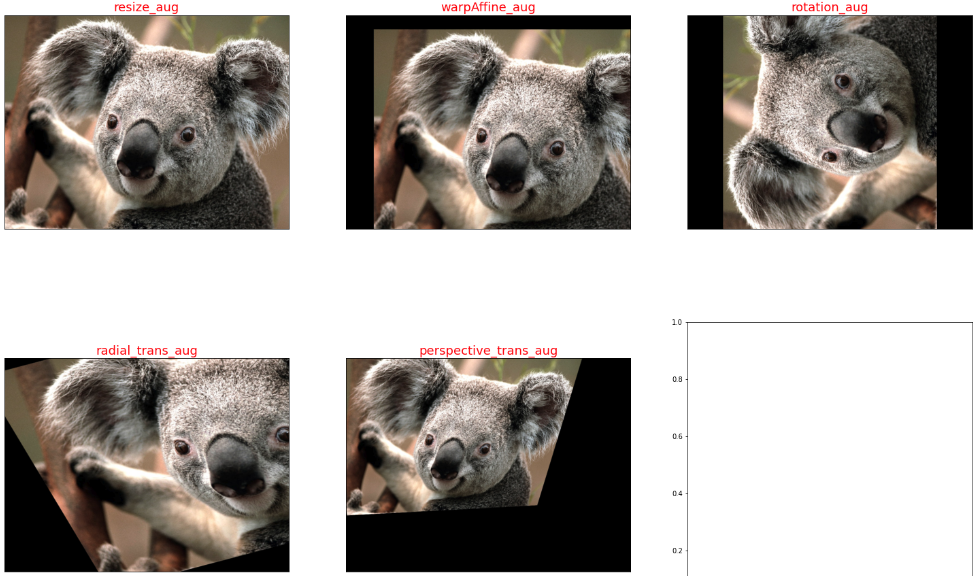### 3.2，pytorch 影象增強

pytorch 框架中，transforms 類提供了 22 個數據增強方法，對應程式碼在 transforms.py 檔案中，它們既可以對 PIL Image 也能對 torch.*Tensor 資料型別進行增強。

api 的詳細介紹可以參考官網文件-Transforming and augmenting images。本章只對 transforms 的 22 個方法進行簡要介紹和總結。

1，裁剪-Crop

• 中心裁剪: transforms.CenterCrop
• 隨機裁剪: transforms.RandomCrop
• 隨機長寬比裁剪: transforms.RandomResizedCrop
• 上下左右中心裁剪: transforms.FiveCrop
• 上下左右中心裁剪後翻轉: transforms.TenCrop

2，翻轉和變換-Flip and Rotations

• 依概率 p 水平翻轉:transforms.RandomHorizontalFlip(p=0.5)
• 依概率 p 垂直翻轉:transforms.RandomVerticalFlip(p=0.5)
• 隨機旋轉:transforms.RandomRotation

3，影象變換

• resize: transforms.Resize
• min-max Normalization: 對應 torchvision.transforms.ToTensor() 方法
• zero-mean Normalization: 對應 torchvision.transforms.Normalize() 方法
• 修改亮度、對比度和飽和度:transforms.ColorJitter
• 轉灰度圖: transforms.Grayscale
• 線性變換: transforms.LinearTransformation()
• 仿射變換: transforms.RandomAffine
• 依概率 p 轉為灰度圖: transforms.RandomGrayscale
• 將資料轉換為 PILImage: transforms.ToPILImage
• transforms.Lambda: Apply a user-defined lambda as a transform.

4，對 transforms 操作，使資料增強更靈活

• transforms.RandomChoice(transforms): 從給定的一系列 transforms 中選一個進行操作
• transforms.RandomApply(transforms, p=0.5): 給一個 transform 加上概率，依概率進行操作
• transforms.RandomOrder: 將 transforms 中的操作隨機打亂

python

# 為了節省空間，這裡不再列出匯入相應庫的程式碼和show_images函式

img_PIL = Image.open('astronaut.jpeg') print(img_PIL.size)

# properly show that the image can be both transformed and not transformed!

torch.manual_seed(0)

# size 引數: desired output size.

resized_imgs = [transforms.Resize(size=size)(orig_img) for size in (30, 50, 100, orig_img.size)] show_images(resized_imgs, 1, 4)### 3.3，imgaug 影象增強

imgaug 是一個用於機器學習實驗中影象增強的庫。 它支援廣泛的增強技術，允許輕鬆組合這些技術並以隨機順序或在多個 CPU 核心上執行它們，具有簡單而強大的隨機介面，不僅可以增強影象，還可以增強關鍵點/地標、邊界框、 熱圖和分割圖。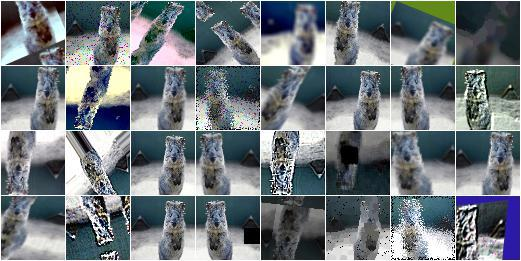imgaug 的影象增強方法如下所示。

• Basics
• Keypoints
• Bounding Boxes
• Heatmaps